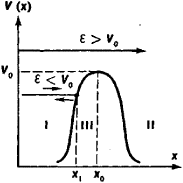# Potential Barrier

(redirected from Finite potential barrier (QM))
Also found in: Wikipedia.

## potential barrier

[pə′ten·chəl ′bar·ē·ər]
(physics)
The potential in a region in a field of force where the force exerted on a particle is such as to oppose the passage of the particle through the region. Also known as barrier; potential hill.
McGraw-Hill Dictionary of Scientific & Technical Terms, 6E, Copyright © 2003 by The McGraw-Hill Companies, Inc.
The following article is from The Great Soviet Encyclopedia (1979). It might be outdated or ideologically biased.

## Potential Barrier

the spatially bounded region of high potential energy of a particle in a field of force on both sides of which the potential energy decreases quite abruptly. The potential barrier corresponds to the repulsive force.

A potential barrier of simple form is illustrated in Figure 1 for the case of linear motion of a particle along the x-axis. When x = x0, the potential energy V(x) assumes a maximum value V0, which is called the height of the potential barrier. A potential barrier divides space into two regions (I and II), in which the potential energy of the particle is less than that in the region (III) within the potential barrier.

In classical mechanics, the passage of a particle through a potential barrier is possible only when the particle’s total energy ℰ—kinetic energy plus potential energy—exceeds the height of the barrier, that is, when ℰ≥ V0. If, however, the energy of the particle is insufficient to surmount the barrier, ℰ< V0, then the particle, moving from left to right, stops upon reaching the barrier at xi and moves in the opposite direction. The potential barrier is a sort of opaque wall or barrier, hence its name, for particles with energy less than its height.Figure 1

In quantum mechanics, in contrast to classical mechanics, the passage of particles with energy less than V0 through the potential barrier is possible. This phenomenon is called the tunnel effect. It also happens that particles with energy greater than V0 are sometimes reflected from the barrier. These peculiarities of the behavior of particles in quantum physics are directly related to the wave-corpuscle nature of subatomic particles. The tunnel effect is significant only in systems having microscopic dimensions and masses. The narrower the potential barrier and the smaller the difference between the height of the barrier and the total energy of the particle, the greater the probability of tunneling.